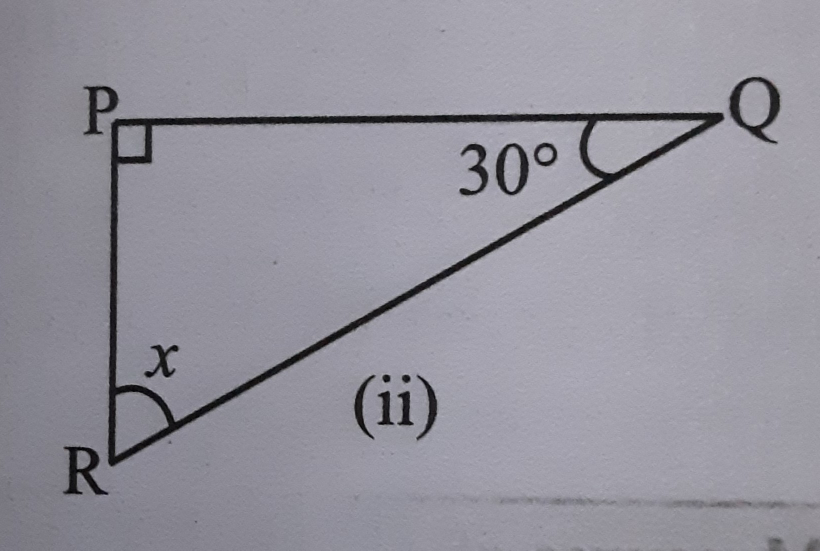"
">

# Find the value of x."

Given: A right-angle triangle with angles x and 30.

To find: We have to find the value of x.

Solution:

We know that in a triangle sum of all the angles is equal to 180o.

∠PQR + ∠QPR + ∠PRQ = 180o

30o + 90o + x = 180o

120o + x = 180o

x = 180o - 120o

x = 60o

Updated on: 10-Oct-2022

26 Views